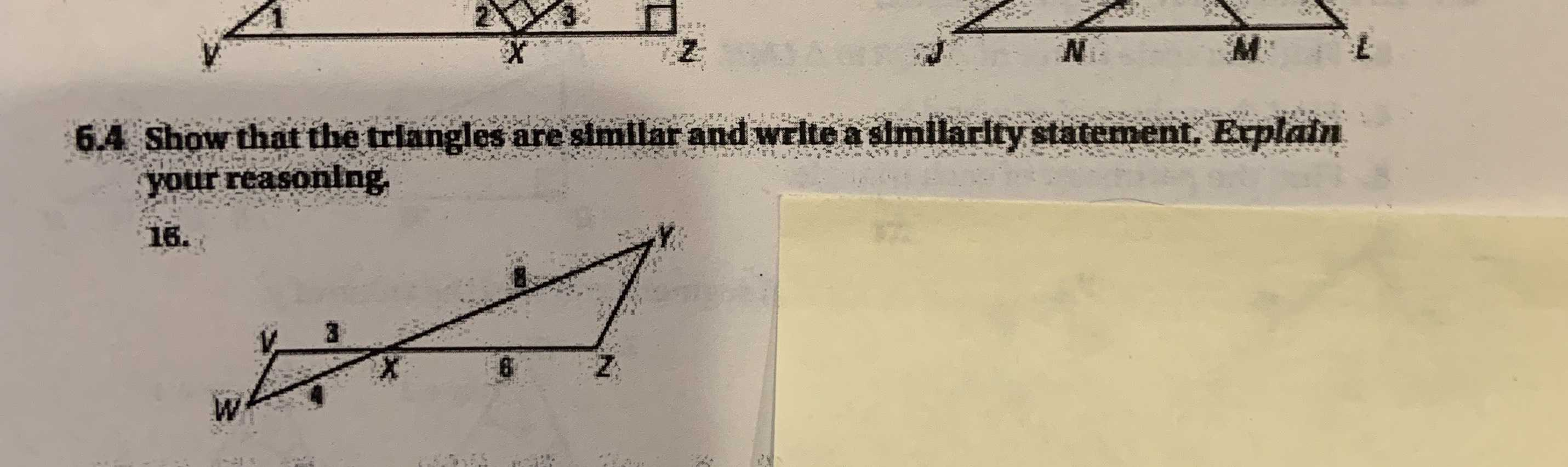### Still have math questions?$$\frac{VX}{WX}= \frac{ZX}{YX}\\\angle VXW= \angle ZXY$$